# Similar Triangles For CAT

One of the most important concepts in the geometry topic is similar triangles. The concept of similar triangles is repeated in the CAT paper every year. Apart from the CAT exam, this concept is also crucial for any competitive exam.

There are even various applications of similar triangles which include checking the stability of bridges, determining heights of structures, etc. Here, the concept of similar triangles is explained in an easy way to help the CAT aspirants prepare this concept more effectively and be able to solve the related questions effectively in the exam.

### What are Similar Triangles?

Two or more triangles are said to be similar if their corresponding angles and sides are congruent and in proportion respectively. In simple words, those triangles which have the same shape, even if the sides and angles are scaled and/ or rotated are called similar triangles. It should be noted that a pair of congruent triangles is always similar.

### Properties of Similar Triangles:

There are two main properties of similar triangles. They are:

• Corresponding angles of two similar triangles are always congruent.
• All the corresponding sides of two similar triangles are always in the same proportion.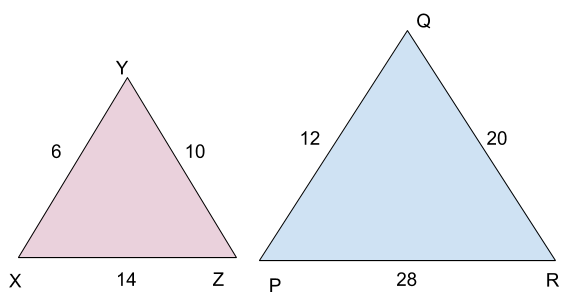In

$$\begin{array}{l}\bigtriangleup\end{array}$$
XYZ and
$$\begin{array}{l}\bigtriangleup\end{array}$$
PQR,
$$\begin{array}{l}\angle\end{array}$$
X =
$$\begin{array}{l}\angle\end{array}$$
P and
$$\begin{array}{l}\frac{XY}{PQ}= \frac{XZ}{PR}= \frac{YZ}{QR}\end{array}$$

### Similarity Tests of Triangles

Two triangles are similar if they fulfil any of the following criteria. With these tests, the similarity of two triangles can be easily checked and related questions can be easily solved.

1. SSS Similarity
2. SAS Similarity
3. AA Similarity
4. HL Similarity

These similarity theorems are discussed in detail below.

• #### SSS Similarity

The Side-Side-Side (SSS) similarity means that two triangles are similar if the lengths of the corresponding sides of two different triangles are in proportion. An example is given below to illustrate this in a better way.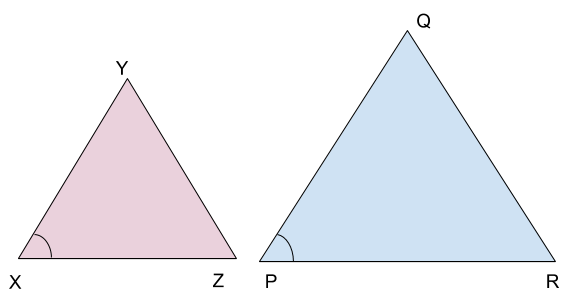In

$$\begin{array}{l}\bigtriangleup\end{array}$$
XYZ and
$$\begin{array}{l}\bigtriangleup\end{array}$$
PQR, the sides are in proportion i.e.,

$$\begin{array}{l}\frac{XY}{PQ}= \frac{YZ}{QR}= \frac{ZX}{RP}\end{array}$$

Thus, it can be said that

$$\begin{array}{l}\bigtriangleup\end{array}$$
XYZ and
$$\begin{array}{l}\bigtriangleup\end{array}$$
PQR are similar angles. It is also crucial to note that
$$\begin{array}{l}\angle\end{array}$$
X=
$$\begin{array}{l}\angle\end{array}$$
P,
$$\begin{array}{l}\angle\end{array}$$
Y=
$$\begin{array}{l}\angle\end{array}$$
Q and
$$\begin{array}{l}\angle\end{array}$$
Z =
$$\begin{array}{l}\angle\end{array}$$
R.

• #### SAS Similarity

In Side-Angle-Side (SAS) similarity, two triangles are similar if any two sides of the triangles are in proportion and the angle between those sides are equal. An example is illustrated below for a better understanding of SAS property.The above-given triangles are said to be similar if:

$$\begin{array}{l}\frac{XY}{PQ}= \frac{XZ}{PR}\end{array}$$
and,
$$\begin{array}{l}\angle\end{array}$$
YXZ =
$$\begin{array}{l}\angle\end{array}$$
QPR.

• #### AA Similarity

This stands for Angle-Angle (AA) similarity. Two triangles are similar if at least two angles of one triangle are equal to any two angles of the other triangle. It is important to note that if two angles are equal for two different triangles, the third one also has to be equal (as total angles in a triangle are 180 degrees). The following diagram illustrates AA similarity.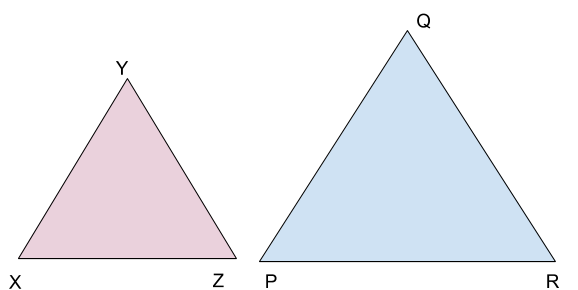$$\begin{array}{l}\bigtriangleup\end{array}$$
XYZ and
$$\begin{array}{l}\bigtriangleup\end{array}$$
PQR are similar by A-A similarity if either two of the angles in
$$\begin{array}{l}\bigtriangleup\end{array}$$
XYZ is equal to either of the two angles in
$$\begin{array}{l}\bigtriangleup\end{array}$$
PQR. From the properties of triangles, it can also be said that the third angle will also be equal as previously mentioned. Thus, this similarity is also called AAA similarity.

• #### HL Similarity

From Hypotenuse-Leg (HL) Similarity, two right triangles are similar if their corresponding sides are equal. This is illustrated below using the following diagram.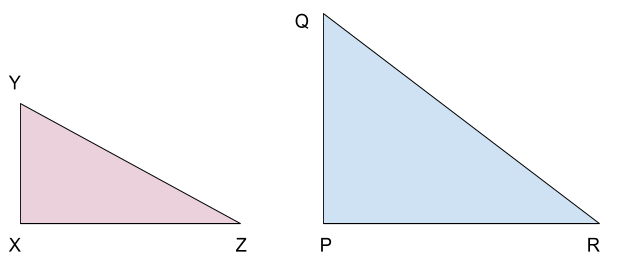$$\begin{array}{l}\bigtriangleup\end{array}$$
XYZ and
$$\begin{array}{l}\bigtriangleup\end{array}$$
PQR are similar if
$$\begin{array}{l}\frac{YZ}{QR}= \frac{YX}{QP}\end{array}$$

#### An example question is given here on similar triangles.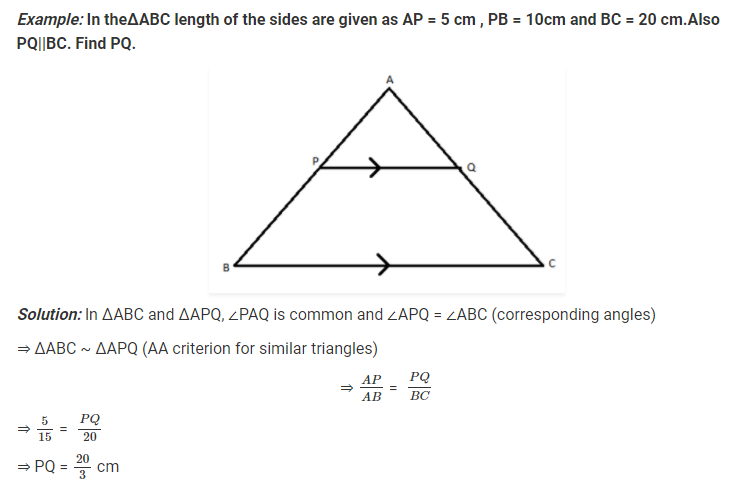Questions related to similar triangles are quickly solvable and scoring. It is always suggested to prepare this topic properly and attempt the related questions efficiently. Learning all the similarity conditions and practising different variations of questions is extremely important to be able to solve all the questions from this topic in the exam.

To learn more important CAT topics, stay tuned with BYJU’S. At BYJU’S, aspiring CAT candidates are also provided with various other study materials, sample papers, mock tests and previous year question papers.